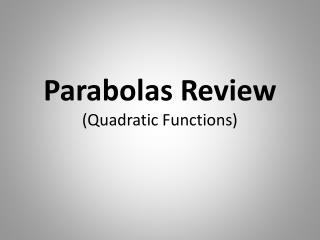DownloadDownload PresentationTélécharger la présentation- - - - - - - - - - - - - - - - - - - - - - - - - - - E N D - - - - - - - - - - - - - - - - - - - - - - - - - - -
##### Presentation Transcript

2. Name Your Forms • In what form is the equation y = 3x2 – 4x + 5 written? • quadratic form

3. Name Your Forms • In what form is the equation y = 3(x – 2)2 + 1 written? • vertex or parabolic form

4. Name Your Forms • In what form is the equation y = x2 + 3 written? • quadratic form • also technically is parabolic or vertex form

5. Name Your Forms • In what form is the equation y = x2 + 3x written? • quadratic form

6. Who’s Who in Parabolic Form • y = a(x – h)2 + k • up/down movement comes from? • k • left/right movement comes from? • h • direction of opening comes from? • a

7. Describe the Functions • y = 3(x – 4)2 + 1 • opens up, vertex (4, 1), stretch by factor of 3, right 4, up 1 • y = -2(x – 3)2 • opens down, vertex (3, 0), stretch by factor of 2, right 3

8. Describe the Functions • y = (x + 2)2 – 3 • opens up, vertex (-2, 3), left 2, down 3 • y = 0.5(x + 1)2 + 4 • opens up, vertex (-1, 4), shrink by factor of .5, left 1, up 4

9. Who’s Who in Quadratic Form • y = ax2 + bx + c • What does the “a” tell you in quadratic form? • direction of opening, stretch or shrink, just like it does in parabolic form

10. Who’s Who in Quadratic Form • y = ax2 + bx + c • What does the “b” tell you in quadratic form? • Nothing! This was a trick question. • “b” is used, however, in the “x of the vertex” formula

11. Who’s Who in Quadratic Form • y = ax2 + bx + c • What does the “c” tell you in quadratic form? • Nothing! Well, that is, unless the question does not have a “bx” term.

12. Who’s Who in Quadratic Form • y = ax2 + c • What does the “c” tell you in THIS version of quadratic form? • Since there is no “bx” term, the “c” tells you the y-intercept.

13. The Vertex Vertex from y = a(x – h)2 + k? (h, k) Vertex from y = ax2 + bx + c? Find x by using –b/2a Find y by plugging in x just found

14. The Vertex Identify the vertices: • y = 2(x + 4)2 – 3? (−4, 3) • y = 0.75(x – 1)2 + 4? (1, 4)

15. The Vertex Identify the vertex: • y = x2 – 6x + 5? • Find x = −b/2a = −(−6)/2(1) = 3 • Solve for y = (3)2 – 6(3) + 5 = −4 • Vertex is (3, −4)

16. The Vertex Identify the vertex: • y = 3x2 + 12x – 4? • Find x = −b/2a = −(12)/2(3) = −2 • Solve for y = 3(−2)2 + 12(−2) − 4 = • Vertex is (−2, −16)

17. The Vertex Identify the vertex: • y = 4x2 + 7? • Remember, there is no “bx” term, so x of vertex will be 0. • Since x of vertex is 0, y will be the constant, or 7. • Vertex is (0, 7)